# Li thresholding¶

In 1993, Li and Lee proposed a new criterion for finding the “optimal” threshold to distinguish between the background and foreground of an image . They proposed that minimizing the cross-entropy between the foreground and the foreground mean, and the background and the background mean, would give the best threshold in most situations.

Until 1998, though, the way to find this threshold was by trying all possible thresholds and then choosing the one with the smallest cross-entropy. At that point, Li and Tam implemented a new, iterative method to more quickly find the optimum point by using the slope of the cross-entropy . This is the method implemented in scikit-image’s `skimage.filters.threshold_li()`.

Here, we demonstrate the cross-entropy and its optimization by Li’s iterative method. Note that we are using the private function _cross_entropy, which should not be used in production code, as it could change.

```import numpy as np
import matplotlib.pyplot as plt
from skimage import data
from skimage import filters
from skimage.filters.thresholding import _cross_entropy

cell = data.cell()
camera = data.camera()
```

First, we let’s plot the cross entropy for the `skimage.data.camera()` image at all possible thresholds.

```thresholds = np.arange(np.min(camera) + 1.5, np.max(camera) - 1.5)
entropies = [_cross_entropy(camera, t) for t in thresholds]

optimal_camera_threshold = thresholds[np.argmin(entropies)]

fig, ax = plt.subplots(1, 3, figsize=(8, 3))

ax.imshow(camera, cmap='gray')
ax.set_title('image')
ax.set_axis_off()

ax.imshow(camera > optimal_camera_threshold, cmap='gray')
ax.set_title('thresholded')
ax.set_axis_off()

ax.plot(thresholds, entropies)
ax.set_xlabel('thresholds')
ax.set_ylabel('cross-entropy')
ax.vlines(optimal_camera_threshold,
ymin=np.min(entropies) - 0.05 * np.ptp(entropies),
ymax=np.max(entropies) - 0.05 * np.ptp(entropies))
ax.set_title('optimal threshold')

fig.tight_layout()

print('The brute force optimal threshold is:', optimal_camera_threshold)
print('The computed optimal threshold is:', filters.threshold_li(camera))

plt.show()
```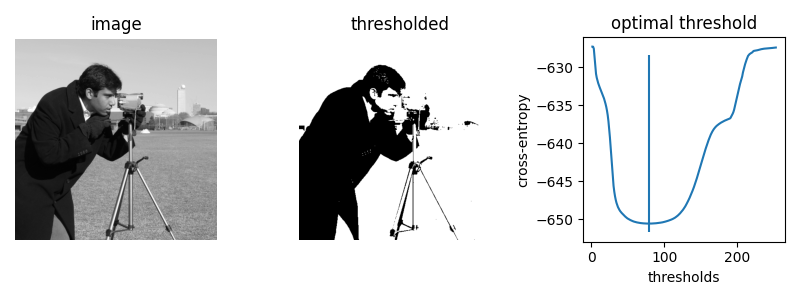```The brute force optimal threshold is: 78.5
The computed optimal threshold is: 78.91288426606151
```

Next, let’s use the `iter_callback` feature of `threshold_li` to examine the optimization process as it happens.

```iter_thresholds = []

optimal_threshold = filters.threshold_li(camera,
iter_callback=iter_thresholds.append)
iter_entropies = [_cross_entropy(camera, t) for t in iter_thresholds]

print('Only', len(iter_thresholds), 'thresholds examined.')

fig, ax = plt.subplots()

ax.plot(thresholds, entropies, label='all threshold entropies')
ax.plot(iter_thresholds, iter_entropies, label='optimization path')
ax.scatter(iter_thresholds, iter_entropies, c='C1')
ax.legend(loc='upper right')

plt.show()
```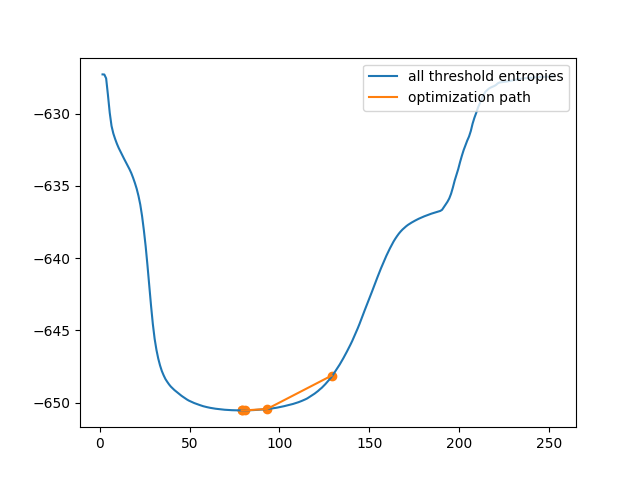```Only 5 thresholds examined.
```

This is clearly much more efficient than the brute force approach. However, in some images, the cross-entropy is not convex, meaning having a single optimum. In this case, gradient descent could yield a threshold that is not optimal. In this example, we see how a bad initial guess for the optimization results in a poor threshold selection.

```iter_thresholds2 = []

opt_threshold2 = filters.threshold_li(cell, initial_guess=64,
iter_callback=iter_thresholds2.append)

thresholds2 = np.arange(np.min(cell) + 1.5, np.max(cell) - 1.5)
entropies2 = [_cross_entropy(cell, t) for t in thresholds]
iter_entropies2 = [_cross_entropy(cell, t) for t in iter_thresholds2]

fig, ax = plt.subplots(1, 3, figsize=(8, 3))

ax.imshow(cell, cmap='magma')
ax.set_title('image')
ax.set_axis_off()

ax.imshow(cell > opt_threshold2, cmap='gray')
ax.set_title('thresholded')
ax.set_axis_off()

ax.plot(thresholds2, entropies2, label='all threshold entropies')
ax.plot(iter_thresholds2, iter_entropies2, label='optimization path')
ax.scatter(iter_thresholds2, iter_entropies2, c='C1')
ax.legend(loc='upper right')

plt.show()
```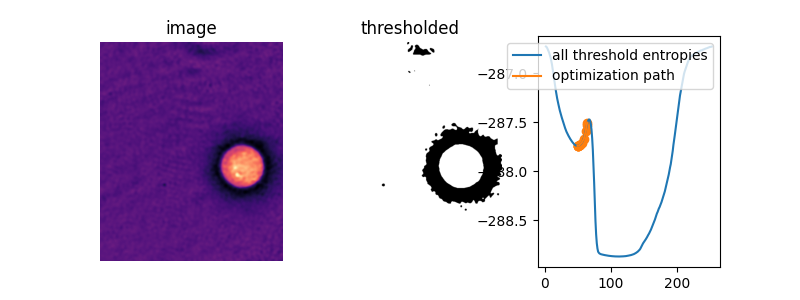In this image, amazingly, the default initial guess, the mean image value, actually lies right on top of the peak between the two “valleys” of the objective function. Without supplying an initial guess, Li’s thresholding method does nothing at all!

```iter_thresholds3 = []

opt_threshold3 = filters.threshold_li(cell,
iter_callback=iter_thresholds3.append)

iter_entropies3 = [_cross_entropy(cell, t) for t in iter_thresholds3]

fig, ax = plt.subplots(1, 3, figsize=(8, 3))

ax.imshow(cell, cmap='magma')
ax.set_title('image')
ax.set_axis_off()

ax.imshow(cell > opt_threshold3, cmap='gray')
ax.set_title('thresholded')
ax.set_axis_off()

ax.plot(thresholds2, entropies2, label='all threshold entropies')
ax.plot(iter_thresholds3, iter_entropies3, label='optimization path')
ax.scatter(iter_thresholds3, iter_entropies3, c='C1')
ax.legend(loc='upper right')

plt.show()
```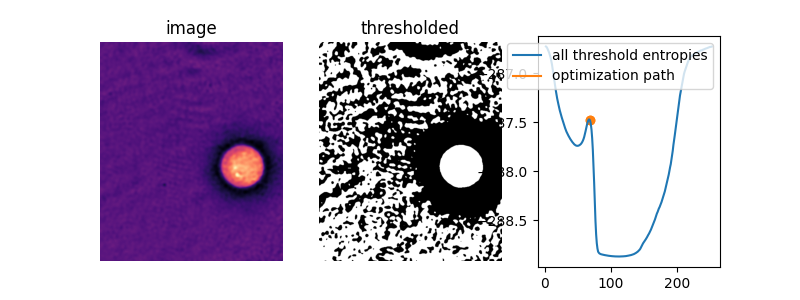To see what is going on, let’s define a function, `li_gradient`, that replicates the inner loop of the Li method and returns the change from the current threshold value to the next one. When this gradient is 0, we are at a so-called stationary point and Li returns this value. When it is negative, the next threshold guess will be lower, and when it is positive, the next guess will be higher.

In the plot below, we show the cross-entropy and the Li update path when the initial guess is on the right side of that entropy peak. We overlay the threshold update gradient, marking the 0 gradient line and the default initial guess by `threshold_li`.

```def li_gradient(image, t):
"""Find the threshold update at a given threshold."""
foreground = image > t
mean_fore = np.mean(image[foreground])
mean_back = np.mean(image[~foreground])
t_next = ((mean_back - mean_fore) /
(np.log(mean_back) - np.log(mean_fore)))
dt = t_next - t
return dt

iter_thresholds4 = []
opt_threshold4 = filters.threshold_li(cell, initial_guess=68,
iter_callback=iter_thresholds4.append)
iter_entropies4 = [_cross_entropy(cell, t) for t in iter_thresholds4]
print(len(iter_thresholds4), 'examined, optimum:', opt_threshold4)

fig, ax1 = plt.subplots()
ax1.plot(thresholds2, entropies2)
ax1.plot(iter_thresholds4, iter_entropies4)
ax1.scatter(iter_thresholds4, iter_entropies4, c='C1')
ax1.set_xlabel('threshold')
ax1.set_ylabel('cross entropy', color='C0')
ax1.tick_params(axis='y', labelcolor='C0')

ax2 = ax1.twinx()
ax2.hlines(, xmin=thresholds2, xmax=thresholds2[-1],
colors='gray', linestyles='dashed')
colors='gray', linestyles='dashed')
ax2.set_ylabel(r'\$\Delta\$(threshold)', color='C3')
ax2.tick_params(axis='y', labelcolor='C3')

fig.tight_layout()

plt.show()
```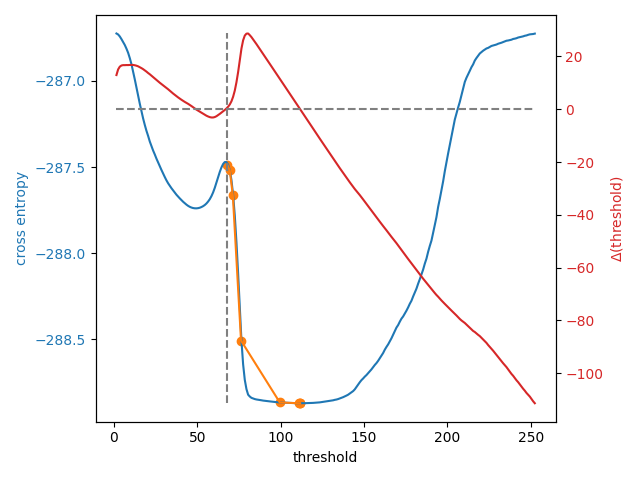```8 examined, optimum: 111.68876119648344
```

In addition to allowing users to provide a number as an initial guess, `skimage.filters.threshold_li()` can receive a function that makes a guess from the image intensities, just like `numpy.mean()` does by default. This might be a good option when many images with different ranges need to be processed.

```def quantile_95(image):
# you can use np.quantile(image, 0.95) if you have NumPy>=1.15
return np.percentile(image, 95)

iter_thresholds5 = []
opt_threshold5 = filters.threshold_li(cell, initial_guess=quantile_95,
iter_callback=iter_thresholds5.append)
iter_entropies5 = [_cross_entropy(cell, t) for t in iter_thresholds5]
print(len(iter_thresholds5), 'examined, optimum:', opt_threshold5)

fig, ax1 = plt.subplots()
ax1.plot(thresholds2, entropies2)
ax1.plot(iter_thresholds5, iter_entropies5)
ax1.scatter(iter_thresholds5, iter_entropies5, c='C1')
ax1.set_xlabel('threshold')
ax1.set_ylabel('cross entropy', color='C0')
ax1.tick_params(axis='y', labelcolor='C0')

plt.show()
```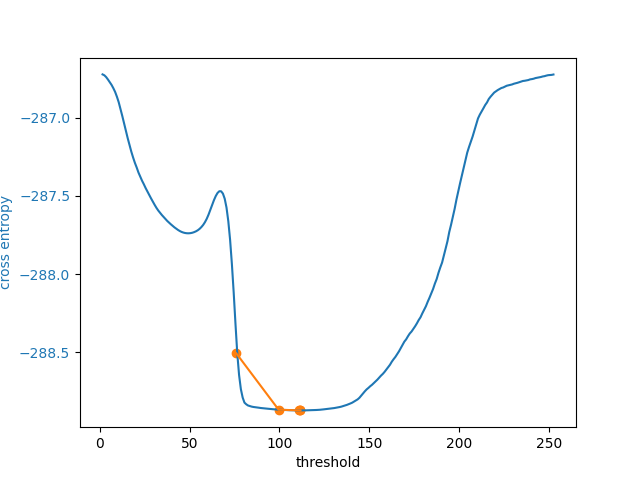```5 examined, optimum: 111.68876119648344
```

Total running time of the script: ( 0 minutes 3.330 seconds)

Gallery generated by Sphinx-Gallery